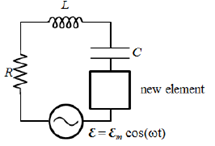# Problem: In a driven, series RLC circuit, the current leads the voltage of the function generator by 60.0° at ω = 40.0 rad/s. If R = 5.00 Ω, what should be added (in series) to the circuit to maximize the power supplied by the function generator? A. 108 mH inductor B. 2.89 mF capacitor C. 8.66 mF capacitor D. 72.2 mH inductor E. 217 mH inductor

###### FREE Expert Solution
86% (146 ratings)
###### Problem Details

In a driven, series RLC circuit, the current leads the voltage of the function generator by 60.0° at ω = 40.0 rad/s. If R = 5.00 Ω, what should be added (in series) to the circuit to maximize the power supplied by the function generator?

A. 108 mH inductor

B. 2.89 mF capacitor

C. 8.66 mF capacitor

D. 72.2 mH inductor

E. 217 mH inductor